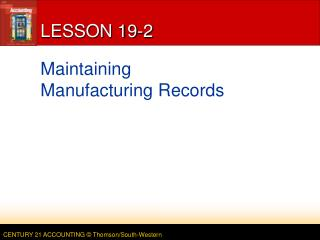DownloadDownload PresentationLESSON 19-2

# LESSON 19-2

Download Presentation## LESSON 19-2

- - - - - - - - - - - - - - - - - - - - - - - - - - - E N D - - - - - - - - - - - - - - - - - - - - - - - - - - -
##### Presentation Transcript

1. LESSON 19-2 Maintaining Manufacturing Records

2. RECORDS FOR MATERIALS page 565 1 2 3 4 • A card should be prepared for each type of material kept in the storeroom • The total value for all the materials ledger cards equals the balance of the materials general ledger account 1. Open a materials ledger card. 2. Record orders placed. 3. Record orders received. 4. Record the new balance. LESSON 19-2

3. RECORDING DIRECT MATERIALS ON A COST SHEET • During the manufacturing process, all costs of making a product must be recorded • All charges for direct materials, direct labor, & factory overhead applied (estimated) for a particular job are recorded on a cost sheet • When direct materials are needed for a job, they are requested from the storeroom using a materials requisition form • A materials requisition form is used to authorize transfer of items from the storeroom to the factory • When materials are issued to the factory from the storeroom, the materials ledger card is updated LESSON 19-2

4. RECORDING DIRECT MATERIALS ON A COST SHEET page 566 1 4 2 1. Open a cost sheet for each job. 2. Prepare a materials requisition. 3. Update the materials ledger card. 4. Record the issuance of direct materials. 3 LESSON 19-2

5. CALCULATING & RECORDING FACTORY OVERHEAD • Factory overhead expenses occur throughout a fiscal period • Factory overhead expenses are not known until the end of a fiscal period • Factory overhead expenses normally are charged to jobs by using an application rate based on a known cost such as direct labor • The estimated amount of factory overhead recorded on cost sheets is called applied overhead LESSON 19-2

6. Estimated No. of Units (Fax Machines) Produced EstimatedDirect Labor Hours per Unit Estimated Salary Rate per Hour EstimatedTotal DirectLabor Cost × × = 10,000 × 8 × \$12.50 = \$1,000,000.00 CALCULATING AND RECORDING FACTORY OVERHEAD page 569 FaxaVision uses direct labor cost as a base unit because there is a close relationship between the amount of direct labor cost & factory overhead costs. FaxaVision estimates next year’s direct labor cost as \$1,000,000.00 • Generally three factors are considered in estimating factory overhead • Amount of factory overhead for the past several fiscal periods • Number of products the factory expects to produce in the next fiscal period • Expected change in unit costs of factory overhead items LESSON 19-2

7. Estimated Factory Overhead Estimated Direct Labor Cost Factory Overhead Applied Rate ÷ = \$800,000.00 ÷ \$1,000,000.00 = .80 or 80% CALCULATING AND RECORDING FACTORY OVERHEAD page 569 FaxaVision’s factory overhead applied rate is 80% of direct labor cost FaxaVision expects to product 10,000 fax machines during the coming year. Considering this volume, previous years’ overhead, and anticipated cost increases, FaxaVision estimates factory overhead as \$800,000 • Estimate the number of base units that will be used in the next fiscal period. • Base units are usually cost items that can be identified easily • Direct labor cost, direct labor hours, & direct materials cost are common bases • A base unit should be selected that most closely relates to actual overhead costs. LESSON 19-2

8. Total Direct Labor Costs (Job No. 791) Factory Overhead Applied Rate Applied Overhead (Job No. 791) × = \$5,024.00 × 80% = \$4,019.20 CALCULATING AND RECORDING FACTORY OVERHEAD page 569 Calculate and record the amount of applied overhead, \$4,019.20, on the cost sheet for Job No. 791 (total direct labor for Job No. 791, \$5,024.00, multiplied by the factory overhead rate, 80%) LESSON 19-2

9. 4 5 Total Costs(Job No. 791) No. of Units Cost per Unit ÷ = \$16,812.50 ÷ 50 = \$336.25 COMPLETING A JOB COST SHEET page 570 1. Total direct materials. 2. Total direct labor. 3. Transfer amounts to Summary columns. 3 4. Calculate applied factory overhead. 5. Determine total job cost. 2 6. Determine cost per unit. 1 6 LESSON 19-2

10. Quantity × Unit Cost = Total Cost FINISHED GOODS LEDGER CARD page 571 2 1 4 3 1. Complete the Manufactured/Received columns. 2. Enter existing inventory balance. 3. Extend data for Job 791. 110 × \$339.30 = \$37,323.00 50 × 336.25 = 16,812.50 \$54,135.50 4. Calculate total cost. LESSON 19-2

11. TERM REVIEW page 572 • applied overhead LESSON 19-2